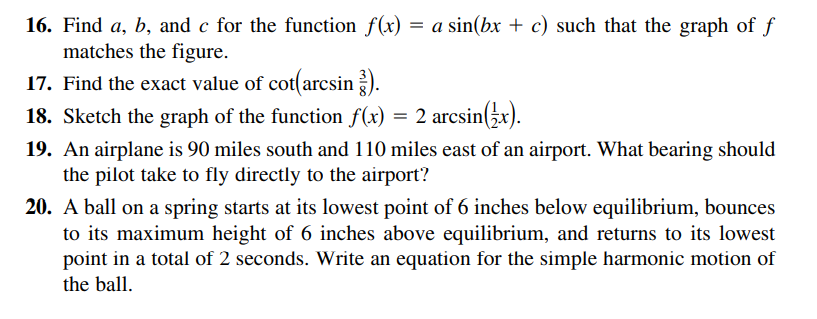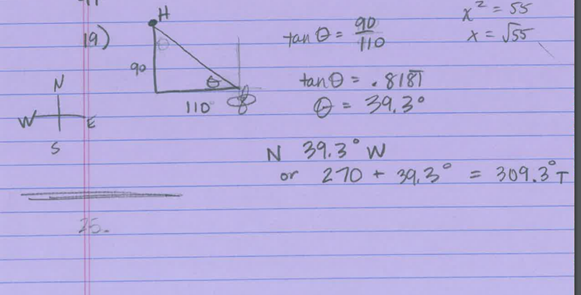### ¿Todavía tienes preguntas de matemáticas?

Pregunte a nuestros tutores expertos
Algebra
Pregunta16. Find $$a , b$$ , and $$c$$ for the function $$f ( x ) = a \sin ( b x + c )$$ such that the graph of $$f$$ matches the figure. 17. Find the exact value of $$\cot ( \arcsin \frac { 3 } { 8 } )$$ . 18. Sketch the graph of the function $$f ( x ) = 2 \arcsin ( \frac { 1 } { 2 } x )$$ . 19. An airplane is $$90$$ miles south and $$110$$ miles east of an airport. What bearing should the pilot take to fly directly to the airport? 20. A ball on a spring starts at its lowest point of $$6$$ inches below equilibrium, bounces to its maximum height of $$6$$ inches above equilibrium, and returns to its lowest point in a total of $$2$$ seconds. Write an equation for the simple harmonic motion of the ball.

$$\frac{\sqrt{55}}{3}$$
19.